PSA6PV2 - Practice Problems---7.1 Lesson 2: Corresponding Parts and Scale Factors (7.G.A.1)
Part A)

The smaller H-shaped polygon is a scaled copy of the larger.

Show one pair of corresponding points between the two polygons. Then, show two pairs of corresponding sides between the polygons.

Consider using colored pencils to highlight corresponding parts or labeling some of the vertices.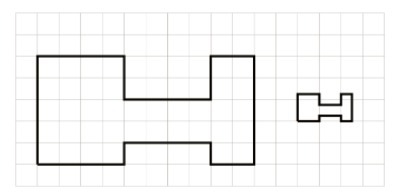Draw your points and sides on paper, take a picture, and upload it using the image upload icon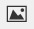If you do not have the ability to upload an image of your work type "drawing is on paper."

Part B)

What scale factor takes the original polygon to its smaller copy?

Type your answer below as a number (example: 5, 3.1, 4 1/2, or 3/2):
Part C)

Explain.

Figure B is a scaled copy of Figure A. Select all of the statements that must be true.

Select all that apply:
Part A)

Polygon B is a scaled copy of Polygon A.

What is the scale factor from Polygon A to Polygon B?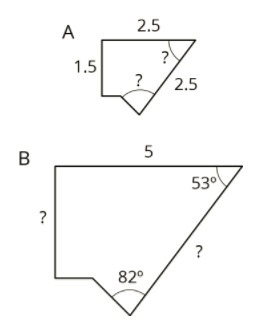Type your answer below as a number (example: 5, 3.1, 4 1/2, or 3/2):
Part B)

Part C)

Find the missing length of the side marked with "C" in Polygon B.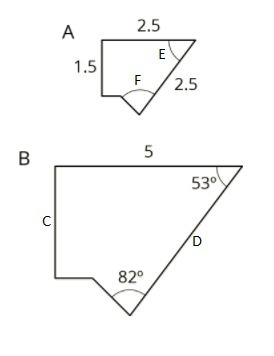Type your answer below as a number (example: 5, 3.1, 4 1/2, or 3/2):
Part D)

Find the missing length of the side marked with "D" in Polygon B.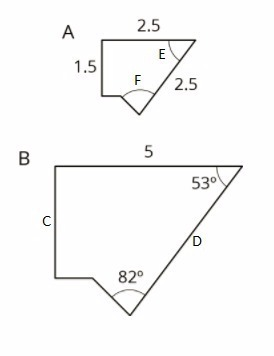Type your answer below as a number (example: 5, 3.1, 4 1/2, or 3/2):
Part E)

Polygon B is a scaled copy of Polygon A.

Determine the measure of the angle marked with "E" in Polygon A.Type your answer below as a number (example: 5, 3.1, 4 1/2, or 3/2):
Part F)

Polygon B is a scaled copy of Polygon A.

Determine the measure of the angle marked with "F" in Polygon A.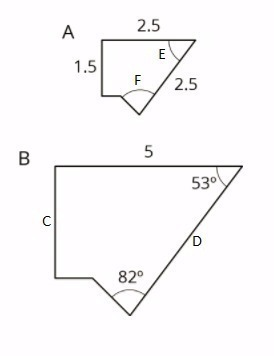Type your answer below as a number (example: 5, 3.1, 4 1/2, or 3/2):

Complete each equation with a number that makes it true.

⋅ ______ 40

Type your answer below as a number (example: 5, 3.1, 4 1/2, or 3/2):

Complete each equation with a number that makes it true.

8 + ______ 40

Type your answer below as a number (example: 5, 3.1, 4 1/2, or 3/2):

Complete each equation with a number that makes it true.

21 ÷ ______ 7

Type your answer below as a number (example: 5, 3.1, 4 1/2, or 3/2):

Complete each equation with a number that makes it true.

21 - ______ 7